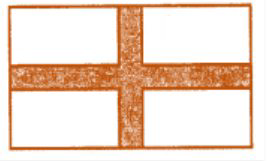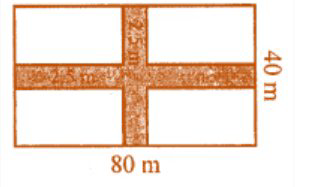Ml aggarwal solutionsOur top 5% students will be awarded a special scholarship to Lido.
Set your child up for success with Lido, book a class today!

A rectangular garden 80 m by 40 m is divided into four equal parts by two cross-paths 2.5 m wide. Find

(i) the area of the cross-paths.

(ii) the area of the unshaded portion.Looking to do well in your science exam ? Learn from an expert tutor. Book a free class!Given,

Length of rectangular garden = 80 m

Width of crossing path 2.5 m

So,

Area of length wise path = 80 × 2.5 = 200 m2

and

Area of breadth wise path = 40 × 2.5 = 100 m2

(i) Total area of both paths

= 200 + 100 – 2.5 × 2.5 m2

= 300 – 6.25 = 293.75 m2

(ii) Area of an unshaded portion

= Area of the garden – Area of paths

= 80 × 40 – 293.75 m2

= 3200 – 293.75 m2

= 2906.25 m2

Our top 5% students will be awarded a special scholarship to Lido.Lido

Courses

Teachers

Book a Demo with us

Syllabus

Science
English
Coding

Terms & Policies

NCERT Question Bank

Maths
Science

Selina Question Bank

Maths
Physics
Biology

Allied Question Bank

Chemistry